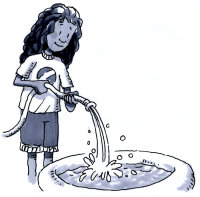### Home > MC2 > Chapter 6 > Lesson 6.2.7 > Problem6-135

6-135.

Beth is filling a small backyard pool with a garden hose. The pool holds $300$ gallons of water. After $15$ minutes the pool is about one-fourth full.

1. Assuming that the water is flowing at a constant rate, about how much water is going into the pool each minute?

First find how many gallons one-fourth of the pool is to find how much has been filled within $15$ minutes.

One-fourth of $300$ gallons is $75$ gallons. Within $15$ minutes, $75$ gallons of water have entered the pool.

To find the rate per minute, divide the amount by the time.
$\frac{75 \text{ gallons}}{15 \text{ minutes}}$

$5$ gallons/minute

1. About how long will it take to fill the pool?

If one-fourth of the pool is filled in $15$ minutes, how long would it take for four-fourths (one whole) of the pool to be filled?